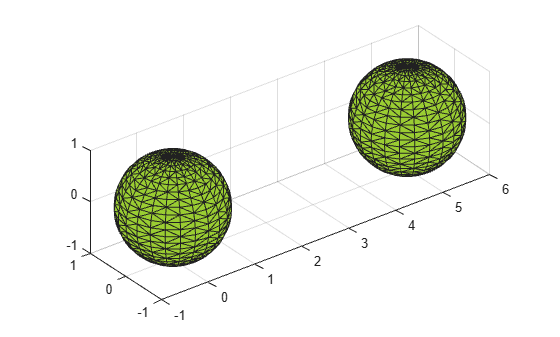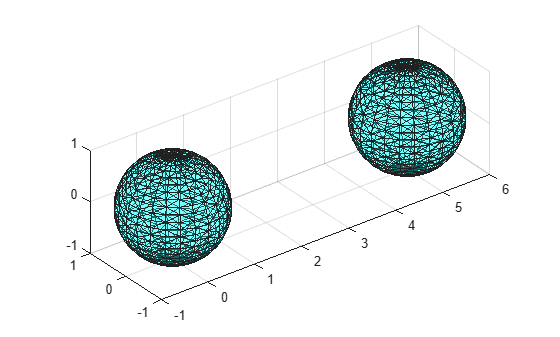# boundaryFacets

Boundary facets of alpha shape

## Syntax

``bf = boundaryFacets(shp)``
``bf = boundaryFacets(shp,RegionID)``
``````[bf,P] = boundaryFacets(___)``````

## Description

example

````bf = boundaryFacets(shp)` returns a matrix representing the facets that make up the boundary of the alpha shape. The facets represent edge segments in 2-D and triangles in 3-D. The vertices of the facets index into the `shp.Points` matrix.```
````bf = boundaryFacets(shp,RegionID)` returns the boundary facets for a region of the alpha shape. `RegionID` is the ID for the region and `1` ≤ `RegionID` ≤ `numRegions(shp)`.```
``````[bf,P] = boundaryFacets(___)``` also returns a matrix of vertex coordinates, `P`, using any of the previous syntaxes. ```

## Examples

collapse all

Create a set of 3-D points.

```[x1, y1, z1] = sphere(24); x1 = x1(:); y1 = y1(:); z1 = z1(:); x2 = x1+5; P = [x1 y1 z1; x2 y1 z1]; P = unique(P,'rows');```

Create and plot an alpha shape using an alpha radius of 1.5.

```shp = alphaShape(P,1.5); plot(shp) axis equal```Compute and plot only the boundary of the alpha shape.

```[tri, xyz] = boundaryFacets(shp); trisurf(tri,xyz(:,1),xyz(:,2),xyz(:,3),... 'FaceColor','cyan','FaceAlpha',0.3) axis equal```## Input Arguments

collapse all

Alpha shape, specified as an `alphaShape` object. For more information, see `alphaShape`.

Example: `shp = alphaShape(x,y)` creates a 2-D `alphaShape` object from the `(x,y)` point coordinates.

ID number for region in alpha shape, specified as a positive integer scalar between `1` and `numRegions(shp)`.

An alpha shape can contain several smaller regions, depending on the point set and parameters. Each of these smaller regions is assigned a unique `RegionID`, which numbers the regions from the largest area or volume to the smallest. For example, consider a 3-D alpha shape with two regions. The region with the largest volume has a `RegionID` of 1, and the smaller region has a `RegionID` of 2.

Example: `shp.RegionThreshold = area(shp,numRegions(shp)-2);` suppresses the two smallest regions in 2-D alpha shape `shp`.

Data Types: `double`

## Output Arguments

collapse all

Boundary facets, returned as a matrix. `bf` is of size `m`-by-`n`, where `m` is the number of boundary facets and `n` is the number of vertices per facet.

Vertex coordinates, returned as a matrix. `P` is of size `N`-by-`dim`, where `N` is the number of points on the boundary of the alpha shape and `dim` is either `2` or `3` (for either a 2-D or 3-D alpha shape).

## Version History

Introduced in R2014b